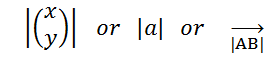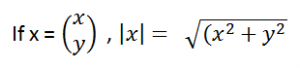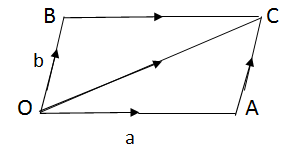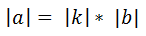Thursday , October 17 2019
ONLINE BOOK STORE (Shop Now)Home / Igcse Mathematics Revision Notes / Vectors (In Two Dimensions)

Vectors (In Two Dimensions)

Vectors have both magnitude and direction.Column Vector:Resultant Vector:

Single Letter in bold. i.e  a.

Position Vector:

Position vectors are also called as end points.Magnitude:To Find the Modulus / Magnitude of a position vector:Addition And Subtraction of Vectors:(Triangular Law of Addition) nose to tail

• The negative sign reverses the directionof a vector.
• The result of a-b is a + – b e. subtracting b is equal to adding the negative of b.

Equal Vectors:

If two line segmants AB  and CD are parallel, in same direction and equal in length, then AB and CD are equal vectors.Parallel Vectors:

Vectors are parallel, if they have the same direction.

Both components of one vector must be in the same ratio to the corresponding components of the parallel vector.

Vector b                        kb (k>0)                         kb (k<0)If a = kb, the vectors a and b are parallel and are in same direction.

Magnitude of a is